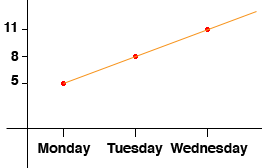Math Central - mathcentral.uregina.caResource Room— glossaries Resource RoomMathematics Glossary - Middle Years Maxine Stinka, Saskatchewan Education, Curriculum and Instruction Note: The definitions included here are those that are used in the Saskatchewan Education document "Mathematics 6-9: A Curriculum Guide for the Middle Level". Various mathematics dictionaries may have different definitions. These definitions are designed to be meaningful to middle level mathematics teachers. Select the first letter of the term that you wish to look for. A | B | C | D | E | F | H | I | J | K | L | M | N | O | P | Q | R | S | T | U | V | W | X | Y | Z L like terms terms that have the same variables raised to the same exponent. e.g. 3x2 and -2x2. linear equation an equation whose graph is a line, that is, an equation that has a degree of one. e.g. y = 3x - 2 linear growth Linear growth means that a quantity grows by the same amount in each step. For example you might have something that is 5 inches long on Monday morning and then 8 inches long on Tuesday morning and then 11 inches long on Wednesday morning and so on. So it is growing by 3 inches a day. If you draw a graph with the days on the horizontal axis and the height in inches on the vertical axis then the points lie on a line. This is why it is called linear growth.line of symmetry a line that divides a figure into two parts, each a mirror image of the other. M mean in statistics, the measure of central tendency calculated by adding all the values and dividing the sum by the number of values. (Often referred to as the average.) median statistical the measure of central tendency that is in the middle when the values are arranged in order of size. If there is an even number of data items, the median is the mean of the middle two. of a triangle a line segment from any vertex of a triangle to the midpoint of the opposite side. mental calculation refers to finding an exact answer mentally (without the use of pencil and paper or calculator.) mixed number a number consisting of a whole number and a fraction. e.g. 5 1/4 mode the value that appears most frequently in a set of data. monomial a number, a variable or a product of numbers and variables. multiplicative inverses(reciprocals) two numbers whose product is 1. Questions or comments about the glossary can be directed to TheCentralizer@MathCentral.uregina.ca or see About Us page for more information.
 Math Central is supported by the University of Regina and the Imperial Oil Foundation.about math central :: site map :: links :: notre site français* Registered trade mark of Imperial Oil Limited. Used under license.